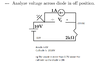# Voltage drop across a diode in a high-amperage circuit

• Engineering
enthdegree
I'm given a strange single-diode circuit where you must find out whether or not the diode is on. See the top diagram in the linked image:

http://i.imgur.com/y1sn3.png

(Current source is indeed 1 Amp, not 1mA as the 2kΩ resistor would suggest. Please do not try to correct the initial question by flipping the diode or whatnot. I have confirmed that this is exactly the question that must be solved.)

So to solve this, I tried examining the circuit as if the diode were open.

I stuck a ground at the positive end of the voltage source for reference (I know it's a weird location but for simple circuit analysis it should be arbitrary, right?) and then looked at the voltage from that point to the positive end of Vd to get Va.

I started again at ground, but this time went towards the negative terminal to get Vb.

I used the voltage difference principle 'V=Va - Vb` to see if the voltage across the gap was greater than 0.7V. It was not.

After asking a few people they say I have a sign error but I cannot find it.

Thanks for the help!

Last edited:

Homework Helper
Gold Member
I believe the diode is ON not OFF. The numbers appear correct but the conclusion at the bottom appears wrong.

If you assume the diode is OFF (as they did) you have 0V on the left hand side of the diode and -2010 on the right hand side. That would imply the diode is ON not OFF.

So then rework the problem assuming the diode is ON... With the +ve of the voltage source at 0V the bottom end of the resistor is at -10V. Voltage across resistor is 9.3V so current flowing through the resistor is 9.3/2k = 4.7mA. Current through diode is 1A + 4.7mA.

#### Attachments

•y1sn3.png
10.2 KB · Views: 422
Last edited:
enthdegree
Ah ha, so you go from the terminals towards the ground, not from the ground towards the terminals!

I was calculating voltage in the wrong direction.

Thank you very much!